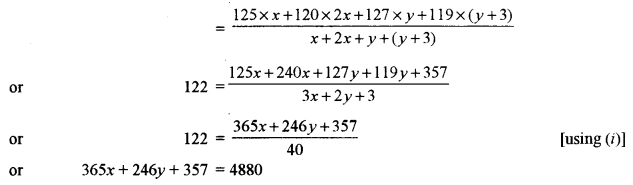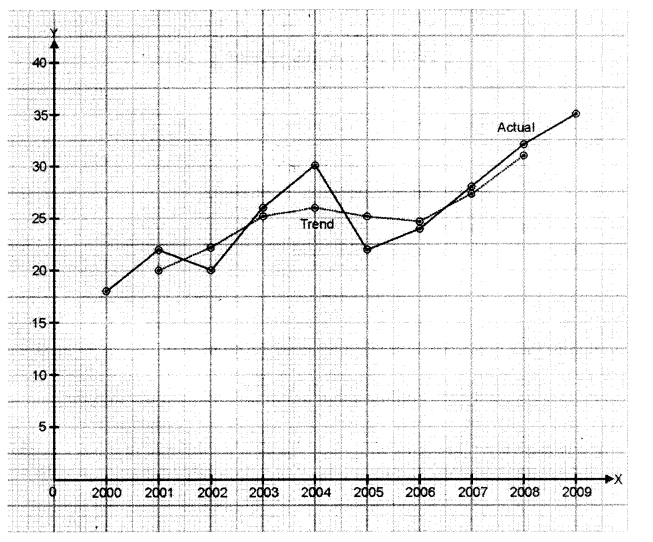## ISC Maths Previous Year Question Paper 2019 Solved for Class 12

Time Allowed: 3 Hours
Maximum Marks: 100

(Candidates are allowed additional 15 minutes for only reading the paper. They must NOT start writing during this time.)

• The Question Paper consists of three sections A, B and C.
• Candidates are required to attempt all questions from Section A and all questions either from Section B or Section C.
• Section A: Internal choice has been provided in three questions of four marks each and two questions of six marks each.
• Section B: Internal choice has been provided in two questions of four marks each.
• Section C: Internal choice has been provided in two questions of four marks each.
• All working, including rough work, should be done on the same sheet as, and adjacent to the rest of the answer.
• The intended marks for questions or parts of questions are given in brackets [ ].
• Mathematical tables and graph papers are provided.

Section – A (80 Marks)

Question 1. [10 × 3]
(i) If the matrix $$\left(\begin{array}{cc}{6} & {-x^{2}} \\ {2 x-15} & {10}\end{array}\right)$$ is symmetric, find the value of x.
(ii) If y – 2x – k = 0 touches the conic 3x2 – 5y2 = 15, find the value of k.
(iii) Prove that $$\frac{1}{2} \cos ^{-1}\left(\frac{1-x}{1+x}\right)=\tan ^{-1} \sqrt{x}$$
(iv) Using L ‘Hospital’s Rule, evaluate:
$$\underset{x \rightarrow \pi / 2}{\mathrm{L}}\left(x \tan x-\frac{\pi}{4} \cdot \sec x\right)$$
(v) Evaluate: $$\int \frac{1}{x^{2}} \sin ^{2}\left(\frac{1}{x}\right) d x$$
(vi) Evaluate: $$\int_{0}^{\pi / 4} \log (1+\tan \theta) d \theta$$
(vii) By using the data $$\overline{x}=25, \overline{y}=30 ; b_{y x}=1.6 \text { and } b_{x y}=0.4$$, find:
(a) The regression equation y on x.
(b) What is the most likely value of y when x = 60?
(c) What is the coefficient of correlation between x and y?
(viii) A problem is given to three students whose chances of solving it are $$\frac { 1 }{ 4 }$$, $$\frac { 1 }{ 5 }$$ and $$\frac { 1 }{ 3 }$$ respectively. Find the probability that the problem is solved.(x) Solve: $$\frac { dy }{ dx }$$ = 1 – xy + y – x
Solution: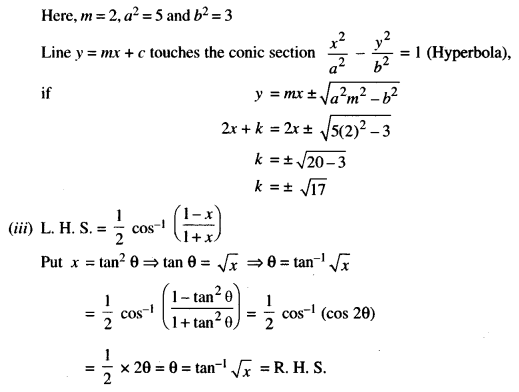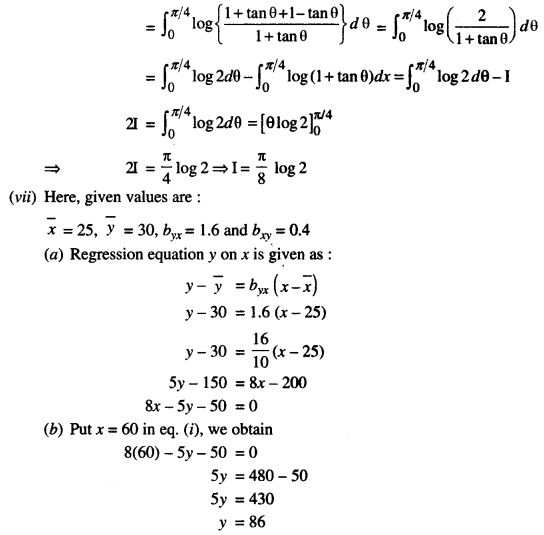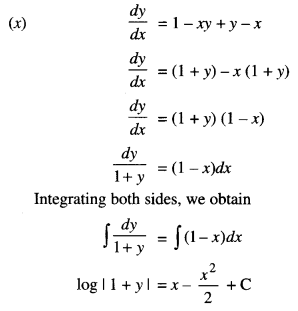Question 2.
(a) Using properties of determinants, prove that:
$$\left|\begin{array}{lll}{a} & {b} & {b+c} \\ {c} & {a} & {c+a} \\ {b} & {c} & {a+b}\end{array}\right|=(a+b+c)(a-c)^{2}$$
(b) Given that: $$A=\left(\begin{array}{ccc}{1} & {-1} & {0} \\ {2} & {3} & {4} \\ {0} & {1} & {2}\end{array}\right) \text { and } B=\left(\begin{array}{ccc}{2} & {2} & {-4} \\ {-4} & {2} & {-4} \\ {2} & {-1} & {5}\end{array}\right)$$, find AB.
Using this result, solve the following system of equation: x – y = 3, 2x + 3y + 4z = 17 and y + 2z = 7
Solution: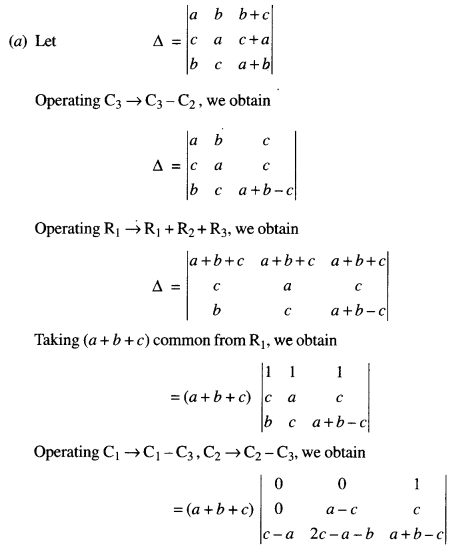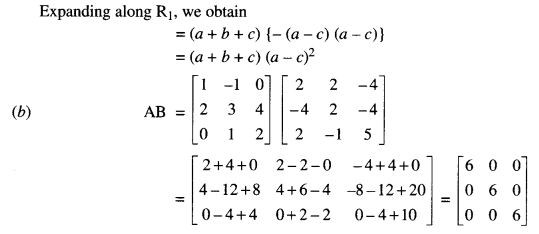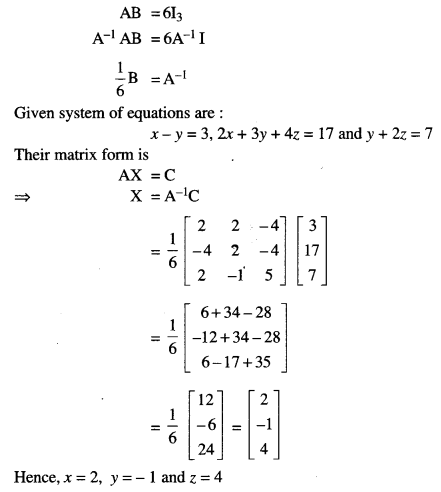Question 3.
(a) Solve the equation for x:
$$\sin ^{-1} x+\sin ^{-1}(1-x)=\cos ^{-1} x, x \neq 0$$
(b) If A, B and C are the elements of Boolean algebra, simplify the expression (A’ + B’) (A + C’) + B’ (B + C). Draw the simplified circuit.
Solution: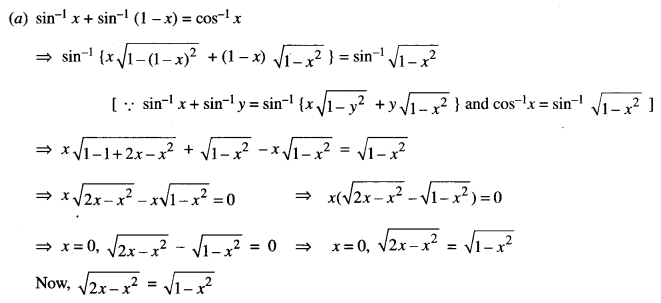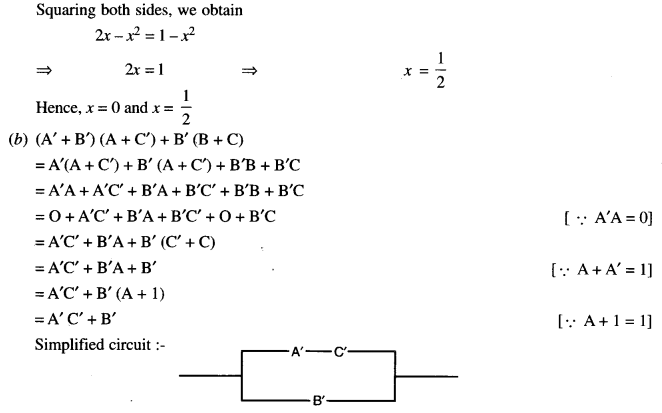Question 4.
(a) Verify Langrange’s mean value theorem for the function: 
f(x) = x (1 – log x) and find the value of ‘c’ in the interval [1, 2]
(b) Find the coordinates of the centre, foci and equation of directrix of the hyperbola x2 – 3y2 – 4x = 8. 
Solution:
(a) Given function ‘f’ is continuous in [1, 2] and differentiable in (1, 2)
f(x) = x (1 – log x) = x – x log x
f'(x) = 1 – x × $$\frac { 1 }{ x }$$ – log x = 1 – 1 – log x
f'(x) = – log x
According to Langrange’s Mean Value Theorem, E a real number c ∈ (1, 2) s.t.,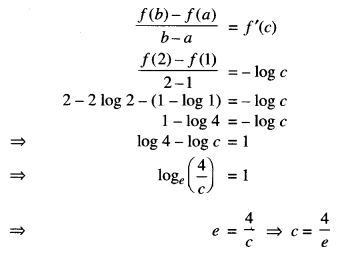Question 5.
(a) If y = cos (sin x), show that: 
$$\frac{d^{2} y}{d x^{2}}+\tan x \frac{d y}{d x}+y \cos ^{2} x=0$$
(b) Show that the surface area of a closed cuboid with square base and given volume is minimum when it is a cube. 
Solution: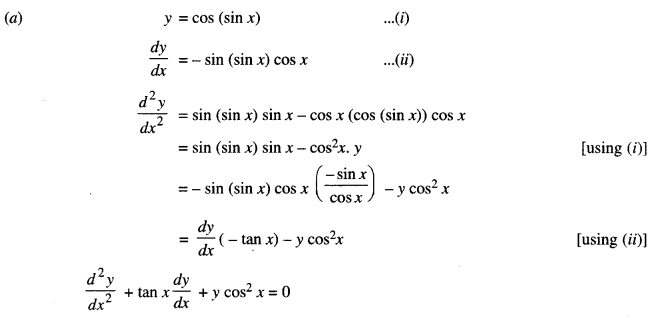(b) Let x be the side of square base of cuboid and other side be y.
Then the volume of a cuboid with square base,
V = x × x × y
⇒ V = x2y
As the volume of the cuboid is given so volume is taken constantly throughout the question, therefore,
$$y=\frac{V}{x^{2}}$$ …….(i)
In order to show that surface area is minimum when the given cuboid is a cube, we have to show S” > 0 and x = y.
Let S be the surface area of cuboid, then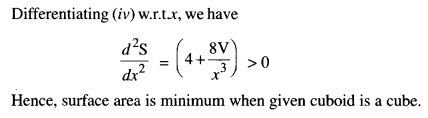Question 6.
(a) Evaluate: $$\int \frac{\sin 2 x}{(1+\sin x)(2+\sin x)} d x$$ 
(b) Draw a rough sketch of the curve y2 = 4x and find the area of region enclosed by the curve and the line y = x. 
Solution: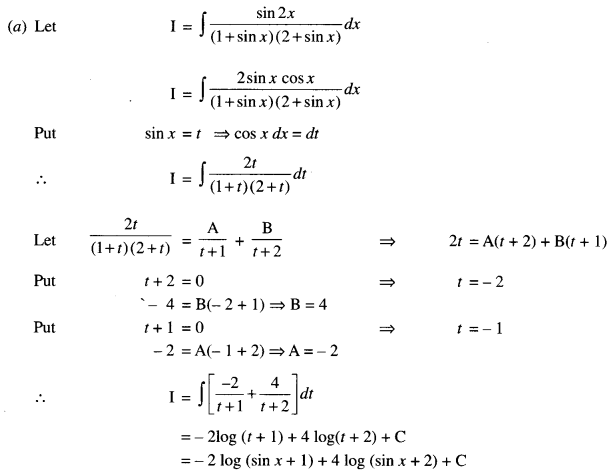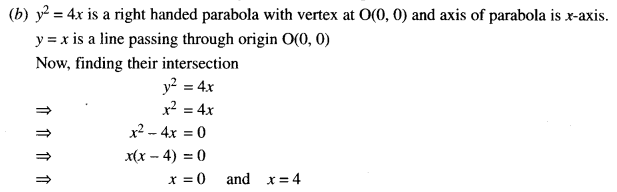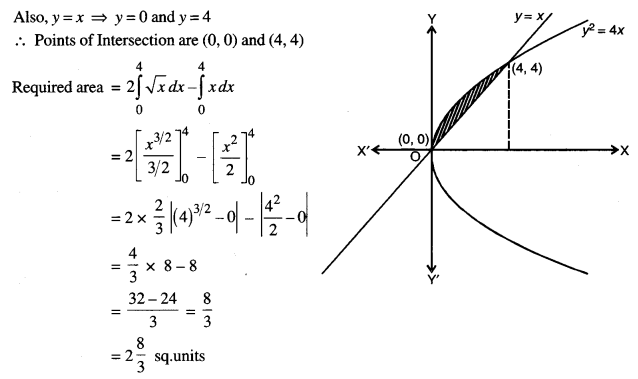Question 7.
(a) Calculate the Spearman’s rank correlation coefficient for the following data and interpret the result: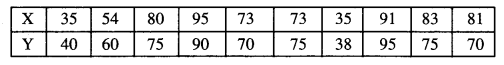(b) Find the line of best fit for the following data, treating x as the dependent variable (Regression equation x on y):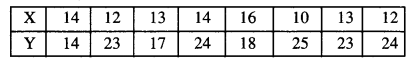Hence, estimate the value of x when y = 16.
Solution:
(a) To determine Spearman’s Rank Correlation: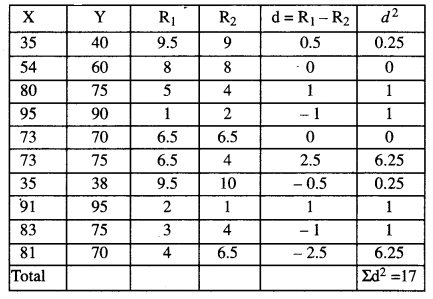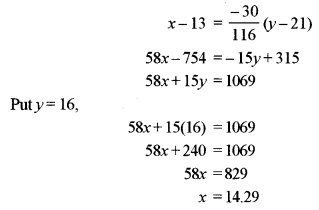Question 8.
(a) In a class of 60 students, 30 opted for Mathematics, 32 opted for Biology and 24 opted for both Mathematics and Biology. If one of these students is selected at random, find the probability that: 
(i) The student opted for Mathematics or Biology.
(ii) The student has opted neither Mathematics nor Biology.
(iii) The student has opted Mathematics but not Biology.
(b) Bag A contains 1 white, 2 blue and 3 red balls. Bag B contains 3 white, 3 blue and 2 red balls. Bag C contains 2 white, 3 blue and 4 red balls. One bag is selected at random and then two balls are drawn from the selected bag. Find the probability that the balls draw n are white and red. 
Solution: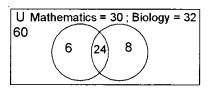U = 60
n(M) = 30
n(B) = 32
n(M ∩ B) = 24
n(M ∪ B) = n(M) + n(B) – n(M ∩ B) = 30 + 32 – 24 = 38
n(M ∪ B)’ = n(∪) – n(M ∪ B) = 60 – 38 = 22
Only Mathematics = n(M) – n(M ∩ B) = 30 – 24 = 6
(i) P(student opted for Mathematics or Biology) = $$\frac { 24 }{ 60 }$$ = $$\frac { 2 }{ 5 }$$
(ii) P(student opted neither Mathematics nor Biology) = $$\frac { 22 }{ 60 }$$ = $$\frac { 11 }{ 30 }$$
(iii) P(student opted Mathematics but not Biology) = $$\frac { 6 }{ 60 }$$ = $$\frac { 1 }{ 10 }$$
(b) Given:
Bag A: 1 white, 2 blue and 3 red balls
Bag B: 3 white, 3 blue and 2 red balls
Bag C: 2 white, 3 blue and 4 red balls
Let B1, B2, B3 and E be the events defined as
B1: Bag A is selected
B2: Bag B is selected
B3: Bag C is selected
And E: 1 white and 1 red ball is drawnQuestion 9.
(a) Prove that locus of z is circle and find its centre and radius if $$\frac{z-i}{z-1}$$ is purely imaginary. 
(b) Solve: (x2 – yx2) dy + (y2 + xy2) dx = 0 
Solution: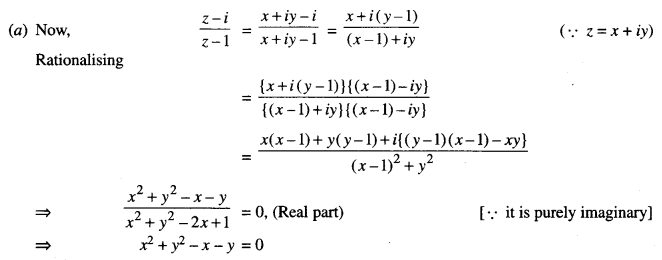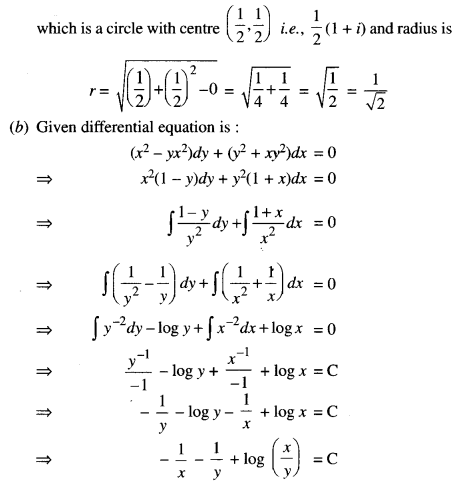Section – B (20 Marks)

Question 10.
(a) If $$\vec{a}, \vec{b}, \vec{c}$$ are three mutually perpendicular vectors of equal magnitude, prove that $$(\vec{a}+\vec{b}+\vec{c})$$ is equally inclined with vectors $$\vec{a}, \vec{b} \text { and } \vec{c}$$. 
(b) Find the value of λ for which the four points with position vectors $$6 \hat{i}-7 \hat{j}, 16 \hat{i}-19 \hat{j}-4 \hat{k}, \lambda \hat{j}-6 \hat{k} \text { and } 2 \hat{i}-5 \hat{j}+10 \hat{k}$$ are coplanar. 
Solution: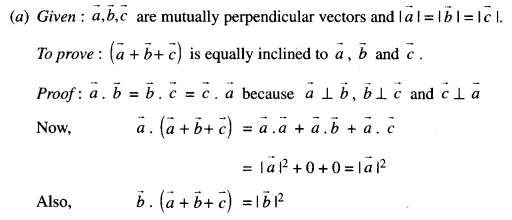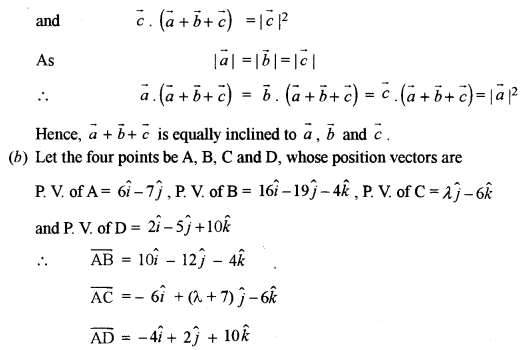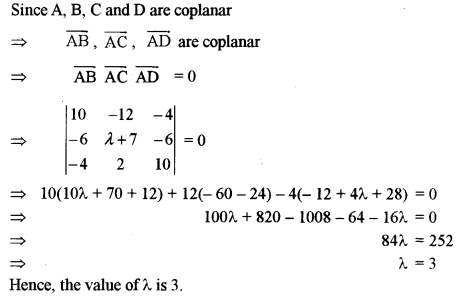Question 11.
(a) Show that the lines $$\frac{x-4}{1}=\frac{y+3}{-4}=\frac{z+1}{7} \text { and } \frac{x-1}{2}=\frac{y+1}{-3}=\frac{z+10}{8}$$ intersect. Find the coordinates of their point of intersection. 
(b) Find the equation of the plane passing through the point (1, -2, 1) and perpendicular to the line joining the points A(3, 2, 1) and B(1, 4, 2). 
Solution:
(a) Given lines are: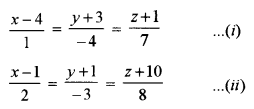Since value of λ in both the cases is same.
Thus, both lines (i) and (ii) intersect each other at a point.
And P(λ + 4, -4λ – 3, 7λ – 1) is P(5, -7, 6).
Hence, the coordinates of the point of intersection are (5, -7, 6).
(b) Here, given that the plane passes through the point (1, -2, 1) and it is perpendicular to the line joining the points A(3, 2, 1) and B(1, 4, 2).
Direction ratios of its normal are < 1 – 3, 4 – 2, 2 – 1 > i.e., < -2, 2, 1 >
Hence, the required equation of the plane is
-2(x – 1) + 2(y + 2) + 1(z – 1) = 0
⇒ -2x + 2 + 2y + 4 + z – 1 = 0
⇒ -2x + 2y + z + 5 = 0
or 2x – 2y – z – 5 = 0

Question 12.
(a) A fair die is rolled. If face 1 turns up, a ball is drawn from Bag A. If face 2 or 3 turns up, a ball is drawn from Bag B. If face 4 or 5 or 6 turns up, a ball is drawn from Bag C. Bag A contains 3 red and 2 white balls, Bag B contains 3 red and 4 white balls and Bag C contains 4 red and 5 white balls. The die is rolled, a Bag is picked up and a ball is drawn. If the drawn ball is red; what is the probability that it is drawn from Bag B? 
(b) An urn contains 25 balls of which 10 balls are red and the remaining green. A ball is drawn at random from the urn, the colour is noted and the ball is replaced. If 6 balls are drawn in this way, find the probability that: 
(i) All the balls are red.
(ii) Not more than 2 balls are green.
(iii) The number of red balls and green balls is equal.
Solution:
(a) Let E1, E2, E3 be the events that a die is thrown and getting 1, 2 or 3 and 4 or 5 or 6 respectively.
$$P\left(E_{1}\right)=\frac{1}{6}, P\left(E_{2}\right)=\frac{2}{6}, P\left(E_{3}\right)=\frac{3}{6}$$
Let A be the event that drawn ball is red
$$P\left(A / E_{1}\right)=\frac{3}{5}, P\left(A / E_{2}\right)=\frac{3}{7}, P\left(A / E_{3}\right)=\frac{4}{9}$$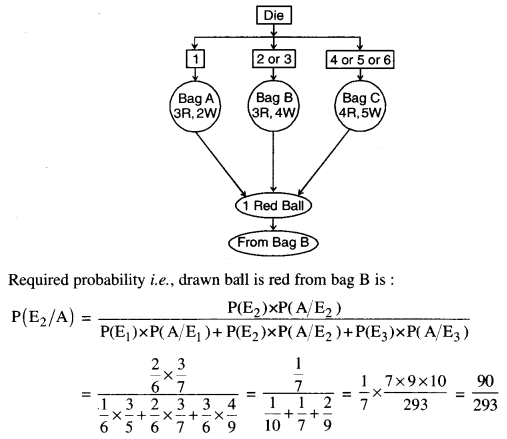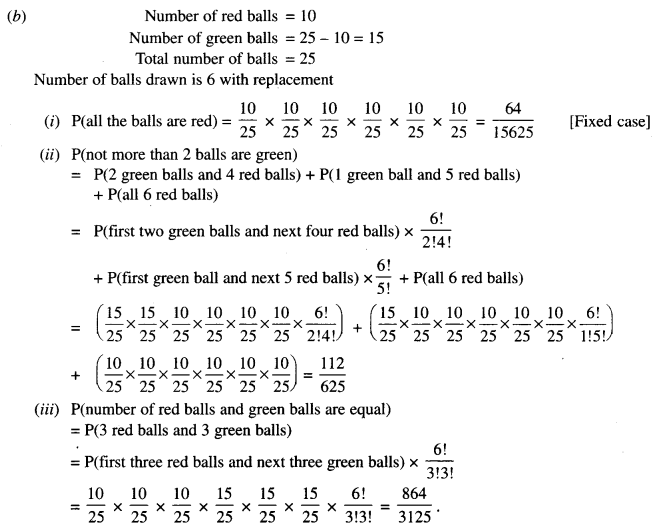Section – C (20 Marks)

Question 13.
(a) A machine costs ₹ 60000 and its effective life is estimated to be 25 years. A sinking fund is to be created for replacing the machine at the end of its life when its scrap value is estimated as ₹ 5000. The price of the new machine is estimated to be 100% more than the price of the present one. Find the amount that should be set aside at the end of each year, out of the profits, for the sinking fund it accumulates at an interest of 6% per annum compounded annually. 
(b) A farmer has a supply of chemical fertilizer of type A which contains 10% nitrogen and 6% phosphoric acid and of type B which contains 5% nitrogen and 10% phosphoric acid. After the soil test, it is found that at least 7 kg of nitrogen and the same quantity of phosphoric acid is required for a good crop. The fertilizer of type A costs ₹ 5.00 per kg and the type B costs ₹ 8.00 per kg. Using Linear programming, find how many kilograms of each type of fertilizer should be bought to meet the requirement and for the cost to be minimum. Find the feasible region in the graph. 
Solution: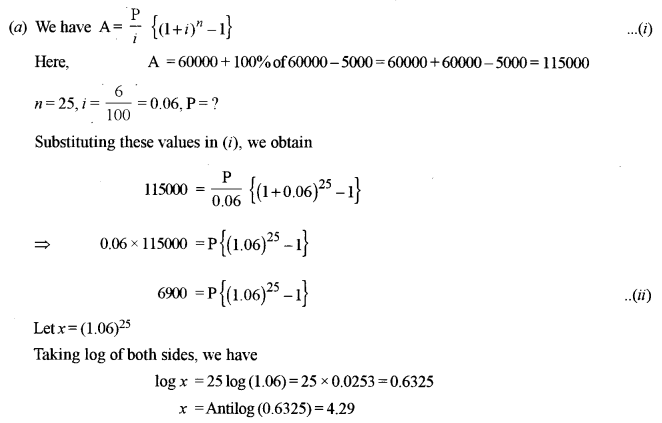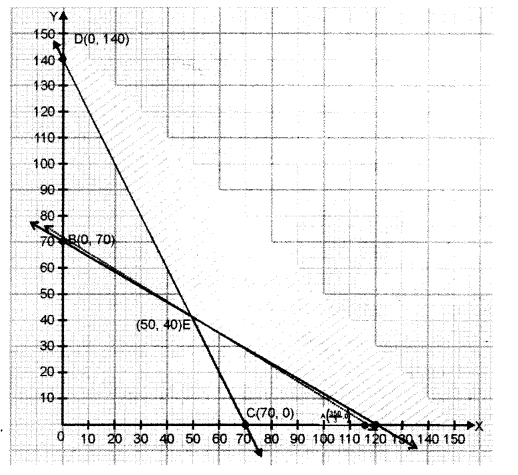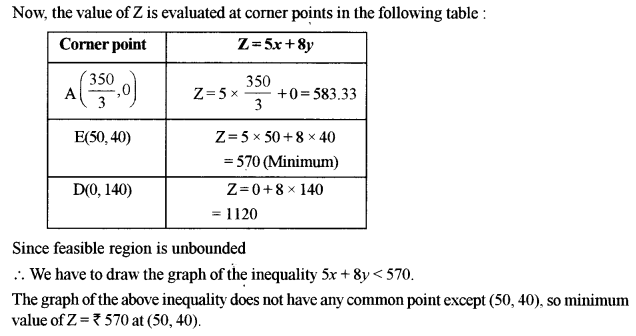Question 14.
(a) The demand for a certain product is represented by the equation $$p=500+25 x-\frac{x^{2}}{3}$$ in rupees, where x is the number of units and p is the price per unit. Find:
(i) Marginal revenue function.
(ii) The marginal revenue when 10 units are sold. 
(b) A bill of ₹ 60000 payable 10 months after the date was discounted for ₹ 57300 on 30th June 2007. If the rate of interest was 11$$\frac { 1 }{ 4 }$$ % per annum, on what date was the bill drawn? 
Solution:
(a) The demand function for a certain product is represented as:
$$p=500+25 x-\frac{x^{2}}{3}$$, p being price per unit
If R be the total revenue for x units, then
$$\mathrm{R}=p \cdot x=500 x+25 x^{2}-\frac{x^{3}}{3}$$
The Marginal Revenue (MR) is given as:
$$\mathrm{MR}=\frac{d(\mathrm{R})}{d x}=500+50 x-x^{2}$$
Marginal Revenue when 10 units are sold i.e., put x = 10
(MR)10 = 500 + 50(10) – (10)2 = 500 + 500 – 100 = 900.
(b) Banker discount (BD) = Face value – Amount received = 60000 – 57300 = ₹ 2700
Now, BD is interested in face value for the remaining periodNow, the bill was drawn on 30 June 2007 for 10 months so legally due date is 3 April 2008.
The bill was enhanced 146 days before discounting days backwards.
April – 3 days
March – 31 days
Feb. – 28 days
Jan. – 31 days
Dec. – 31 days
Nov. – 19 days
Bill was encashed on (30 – 19) = 11th Nov, 2007.

Question 15.
(a) The price relatives and weights of a set of commodities are given below:If the sum of the weights is 40 and the weighted average of price relatives index number is 122, find the numerical values of x and y.
(b) Construct 3 yearly moving averages from the following data and show on a graph against the original data: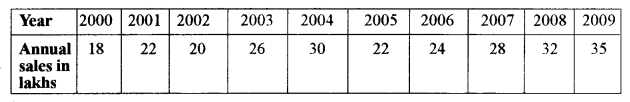Solution:
(a) Given x + 2x + y + (y + 3) = 40
or 3x + 2y = 37 …(i)
and I = the index for the set = 122
We have I = weighted average of price-relatives